# How to model the BESSY cavity with GdfidL v1

Warner Bruns

November 14, 2003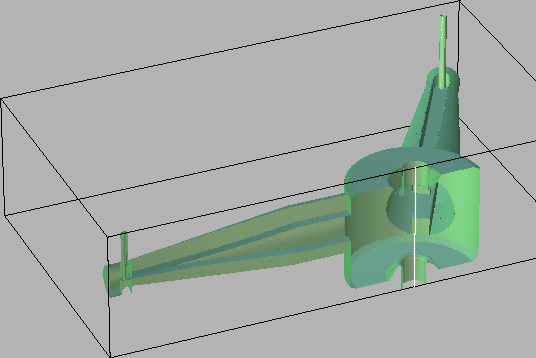# What this paper contains

We got detailed technical data1 of the proposed BESSY cavity.

The technical data are transformed step by step into an inputfile for gd1. Every step is explained in detail. Numerous hints are given, how one effectively models the geometry.

# Recommended usage of gd1

The most effective usage of gd1 is:
• Write an inputfile
• Until gd1 does what you want him to do:
• feed gd1 with the inputfile
• Change the inputfile
If you work with several xterms, this cycle is very fast. Figure 1.1 shows a typical desktop with three xterms. The upper right xterm is a terminal running an editor to change the inputfile, the lower right xterm is used to feed gd1 with the actual inputfile, and a third xterm is used to run another instance of gd1. This second instance of gd1 is used to look-up the syntax of gd1's commands.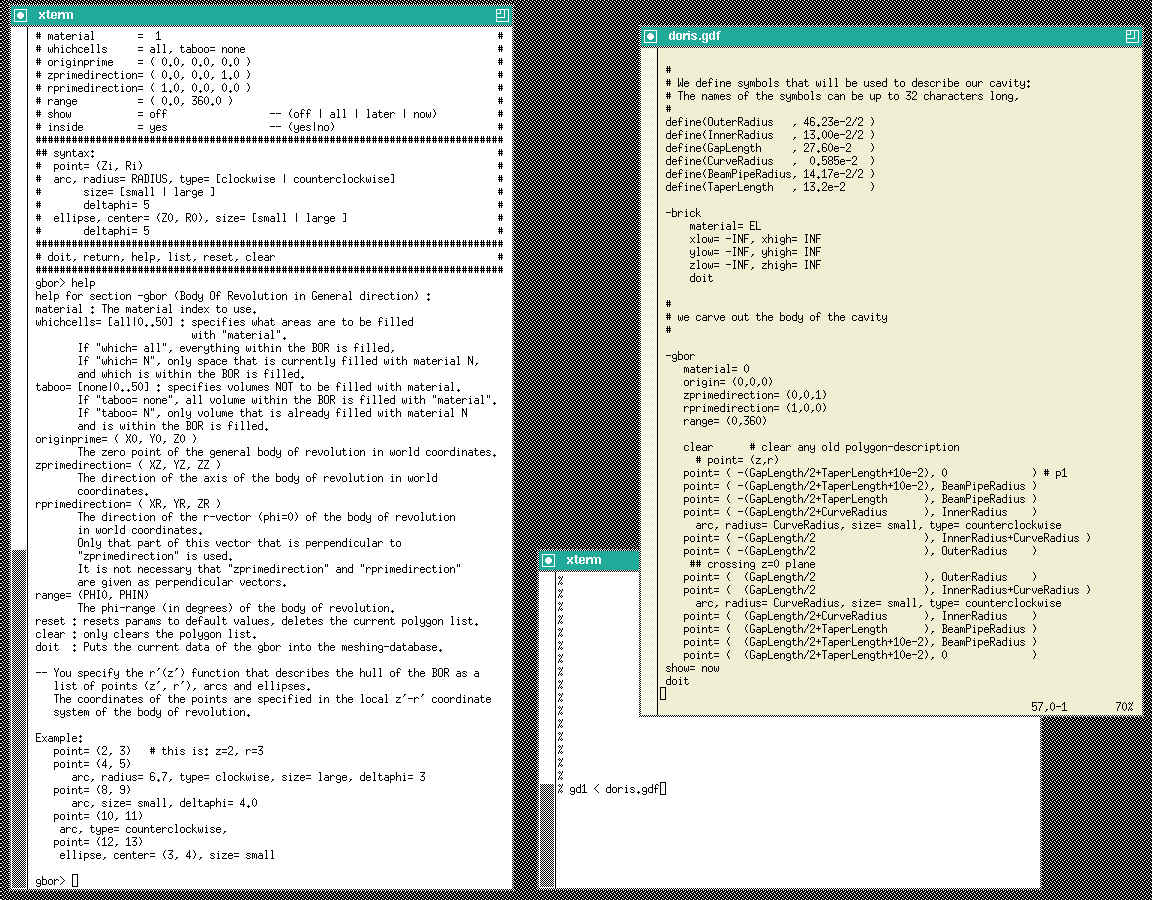# Modelling the geometry

The cavity we want to analyse is essentially a body of revolution. There are three higher order mode (HOM) couplers attached. The hard to model part are the HOM-couplers.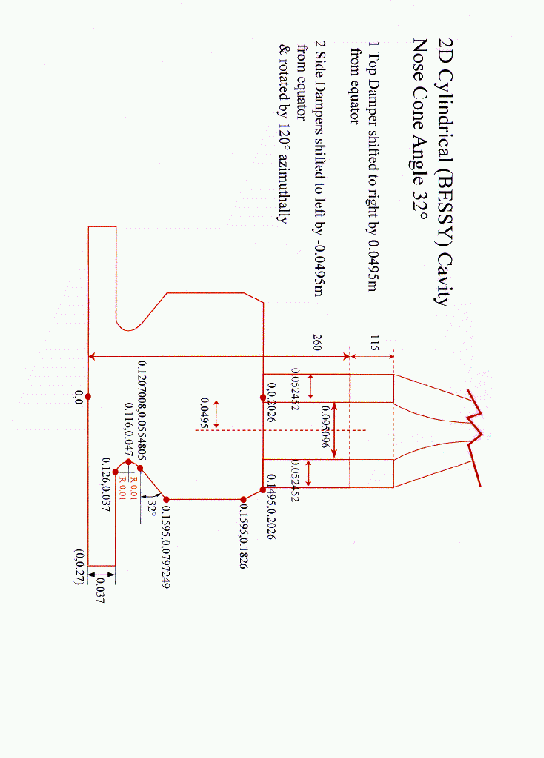In the first step we model the cavity and the beam-pipes. We do this by specifying a polygonal description of the boundary in the r-z-plane. The inputfile that describes the boundary is:

 #
#
define(EL, 1) define(MAG, 2)
define(INF, 1000)

#
# We define symbols that will be used to describe our cavity:
# The names of the symbols can be up to 32 characters long,
#
define(GapLength     , 27.60e-2   )
define(TaperLength   , 13.2e-2    )

########
#
# We fill the universe with metal
#
-brick
material= EL
xlow= -INF, xhigh= INF
ylow= -INF, yhigh= INF
zlow= -INF, zhigh= INF
doit

#
# we carve out the body of the cavity
#

-gbor
material= 0
origin= (0,0,0)
range= (0,360)

clear
#
# the following polygon describes the upper part of the cavity:
#
point= ( -1e-6, 0) # (z,r)
point= ( 0.1+0.27, 0)
point= ( 0.1+0.27, 0.037 )
point= ( 0.126, 0.037)
arc, radius= 0.01, size= small, type= clockwise
point= ( 0.116, 0.047)
arc,
point= ( 0.1207008, 0.0554805 )
point= ( 0.1595, 0.0797249 )
point= ( 0.1595, 0.1836 )
point= ( 0.1495, 0.2027 ) # 0.2027 am 7.ten November 2003
point= ( 0, 0.2027 )
point= ( -1e-6, 0 )

#
# specify the r- and z-direction of the BOR,
# model the upper part.
#
rprime= ( 1, 0, 0 )
zprime= ( 0, 0, 1 ) # Upper part of the cavity
show= later
doit

#
# re-specify the z-direction,
# model the lower part.
#
zprime= ( 0, 0, -1 ) # lower part of the cavity
show= all
doit

-volumeplot
doit

This inputfile can be found as "/usr/local/gd1/Tutorial-Bessy/bessy00.gdf". When we feed this file into gd1 via the command "gd1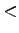bessy00.gdf", we get a desktop similiar to the one shown in figure 1.3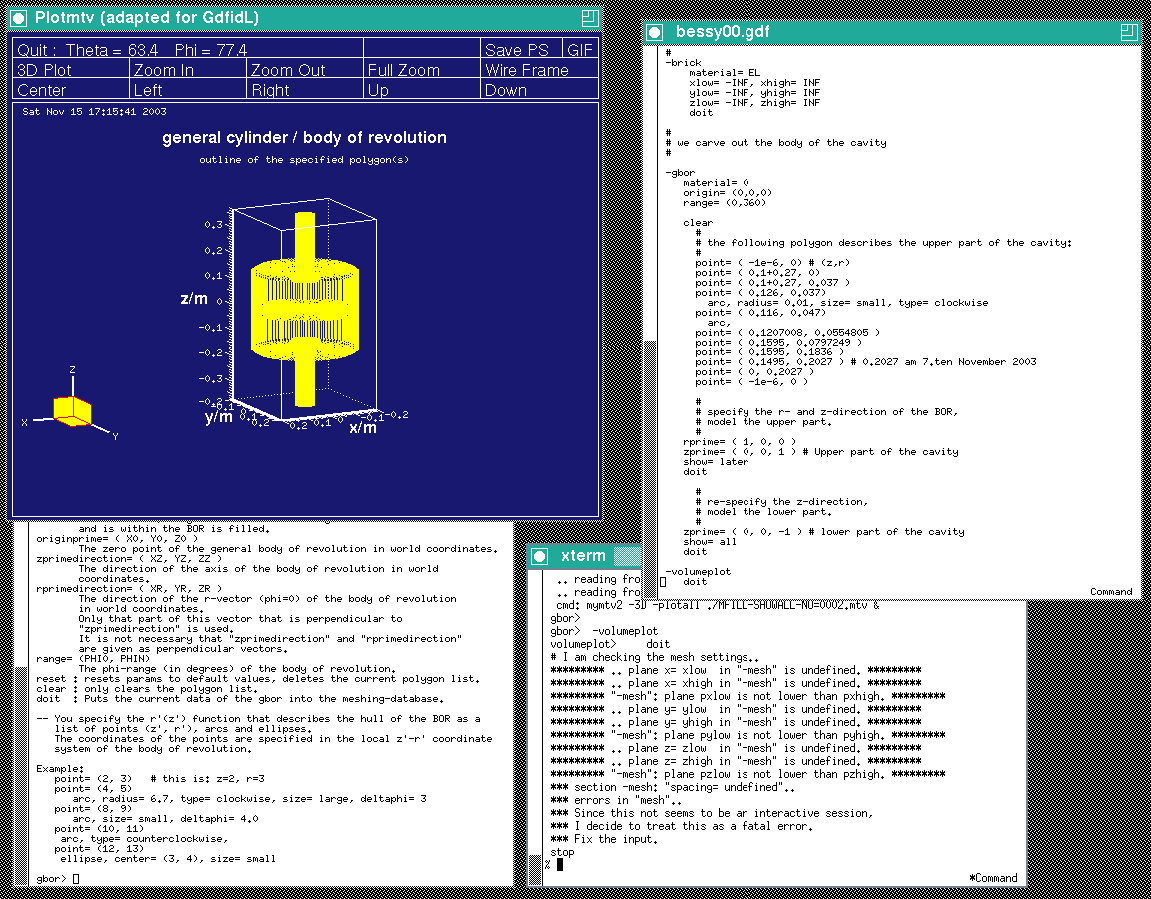gd1 does not what we want, we do not get a "volumeplot", although we requested one. But gd1 gives us a hint what we made wrong:

 gbor>  -volumeplot
volumeplot>     doit
# I am checking the mesh settings..
********* .. plane x= xlow  in "-mesh" is undefined. *********
********* .. plane x= xhigh in "-mesh" is undefined. *********
********* "-mesh": plane pxlow is not lower than pxhigh. *********
********* .. plane y= ylow  in "-mesh" is undefined. *********
********* .. plane y= yhigh in "-mesh" is undefined. *********
********* "-mesh": plane pylow is not lower than pyhigh. *********
********* .. plane z= zlow  in "-mesh" is undefined. *********
********* .. plane z= zhigh in "-mesh" is undefined. *********
********* "-mesh": plane pzlow is not lower than pzhigh. *********
*** section -mesh: "spacing= undefined"..
*** errors in "mesh"..
*** Since this not seems to be an interactive session,
*** I decide to treat this as a fatal error.
*** Fix the input.
stop

When we say "doit" in the section "-volumeplot", gd1 tries to generate the mesh. But in order to generate the mesh, gd1 needs to know
• what the extreme coordinates of the computational volume shall be,
• what the default mesh spacing shall be.
All these informations have to be given to gd1 before a volumeplot is requested. Since gd1 has detected that it is fed by an inputfile and is not used interactively, it stops as soon some essential information is not available. When not run interactively, gd1 also stops when some syntax error is present in the inputfile.

To give gd1 the needed information, we change our inputfile. We insert the following lines somewhere before "-volumeplot":

 ###
### We define the borders of the computational volume,
### and we define the default mesh-spacing.
###
-mesh
pzlow = -(GapLength/2+TaperLength+9e-2)
pzhigh= +(GapLength/2+TaperLength+9e-2)

The so edited inputfile can be found as "/usr/local/gd1/Tutorial-Bessy/bessy01.gdf".

When we feed gd1 with this inputfile (gd1bessy01.gdf) we get a screen similiar to the one shown in figure 1.4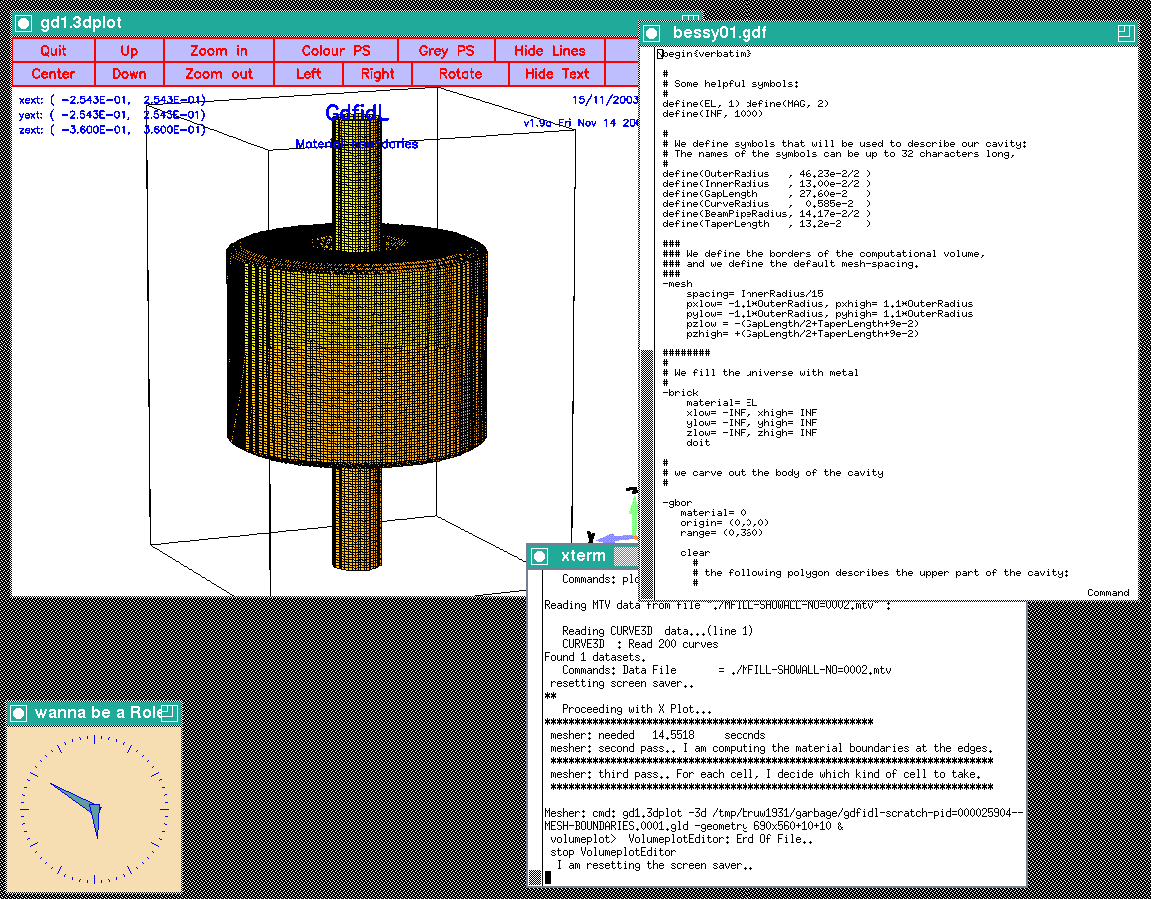# Modelling the real 3D geometry

The real geometry has three higher oder mode (HOM) couplers attached. The couplers consist of a hollow waveguide section and a hollow waveguide to coaxial waveguide adapter. The coaxial waveguide is terminated by a matched load.

The hollow waveguide section is a ridged waveguide where the cross section varies continously. The waveguide is designed such, that at every cross section the cutoff frequency of the fundamental mode is the same.

# Modelling a straight waveguide attached under an arbitrary angle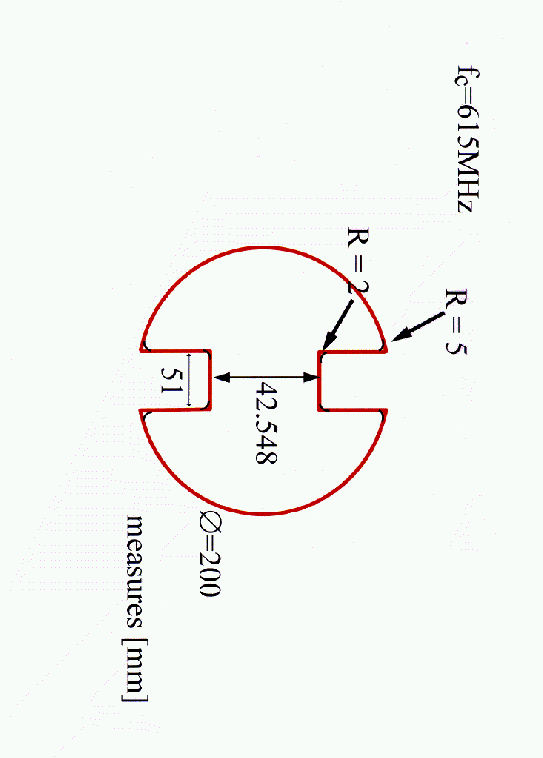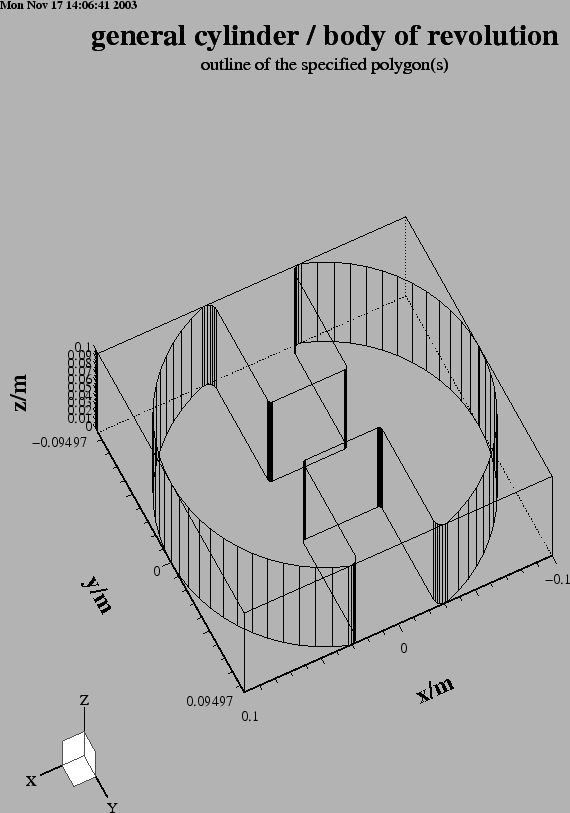The waveguides a general cylinders, where the cross section of the cylinders is some polygon. gd1 can model this directly. We edit our inputfile so that it contains:
 define(GuideR0, 200e-3/2)
define(GuideGap, 42.548e-3)
define(WW, 51e-3)
define(RR2, 2e-3)
define(RR5, 5e-3)

-ggcylinder
material= 0
range= ( 0, 100e-3 )

define(AA, WW/2+RR5)
define(BB, ((GuideR0-RR5)**2 - AA**2)**0.5)
define(THETA, atan2(BB, AA))

clear

# +x, +y
point= ( GuideR0, 0 ) # x', y'
arc, radius= GuideR0, size= small, type= counterclockwise, deltaphi= 5
point= ( GuideR0*cos(THETA), GuideR0*sin(THETA) )
arc, radius= RR5, size= small, type= counterclockwise, , deltaphi= 15
point= (  WW/2   , BB )
point= (  WW/2   , GuideGap/2+ RR2 )
arc, radius= RR2, size= small, type= clockwise
point= (  WW/2-RR2 , GuideGap/2 )

# -x, +y
point= ( -(WW/2-RR2), GuideGap/2 )
arc, radius= RR2, size= small, type= clockwise
point= ( -WW/2   , GuideGap/2+ RR2 )
point= ( -WW/2   , BB )
arc, radius= RR5, size= small, type= counterclockwise, , deltaphi= 15
point= ( -GuideR0*cos(THETA), GuideR0*sin(THETA) )
arc, radius= GuideR0, size= small, type= counterclockwise, deltaphi= 5
point= ( -GuideR0, 0 ) # x', y'

# -x, -y
point= ( -GuideR0, 0 ) # x', y'
arc, radius= GuideR0, size= small, type= counterclockwise, deltaphi= 5
point= ( -GuideR0*cos(THETA), -GuideR0*sin(THETA) )
arc, radius= RR5, size= small, type= counterclockwise, , deltaphi= 15
point= (  -WW/2   , -BB )
point= (  -WW/2   , -(GuideGap/2+ RR2) )
arc, radius= RR2, size= small, type= clockwise
point= (  -(WW/2-RR2), -GuideGap/2 )

# +x, -y
point= (  (WW/2-RR2), -GuideGap/2 )
arc, radius= RR2, size= small, type= clockwise
point= (  WW/2   , -(GuideGap/2+ RR2) )
point= (  WW/2   , -BB )
arc, radius= RR5, size= small, type= counterclockwise, , deltaphi= 15
point= (  GuideR0*cos(THETA), -GuideR0*sin(THETA) )
arc, radius= GuideR0, size= small, type= counterclockwise, deltaphi= 5
point= (  GuideR0, 0 ) # x', y'

show= all
doit


The figure 1.6 shows an outline of the waveguide that this decribes. This waveguide has its axis gowing in z-direction. Our waveguides shall have their axis lying in the x-y-plane, with an angle of 0, 120 and 240 degrees. In order to have the axis of our waveguide direct in the proper direction, we change the values of xprimedirection, yprimedirection. These two vectors define the local x', y' coordinate-system, in which the foorprint of the general cylinder is described. We edit our inputfile:

 -ggcylinder
material= 0
range= ( 0, 100e-3 )

define(PHI, 120*@pi/180 )
xprimedirection= ( cos(PHI-@pi/2), sin(PHI-@pi/2), 0 )
yprimedirection= ( 0, 0, 1 )

The resulting outline is shown in figure 1.7.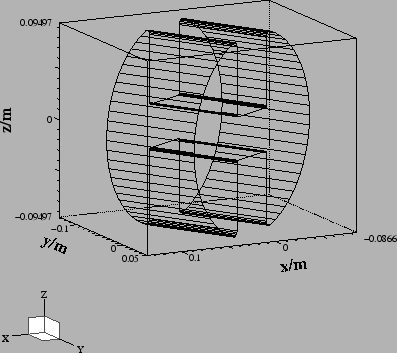## Modeling three straight waveguides

Since we have to model three waveguides, we could edit our inputfile to contain the description of the other waveguides as well. But this is a good opportunity to use a macro.

Anywhere in an inputfile we can define macros. A macro is enclosed between two lines: The first line contains the keyword macro followed by the name of the macro. All lines until a line with only the keyword endmacro are considered the body of the macro. When gd1 or gd1.pp find such a macro, they read it and store the body of the macro in an internal buffer.
Example

   #
# This defines a macro with name 'foo'
#
macro foo
echo I am foo, my first argument is @arg1
echo The total number of arguments supplied is @nargs
endmacro

When gd1 or gd1.pp find a call of the macro, the number of the supplied arguments is assigned to the variable @nargs, and the variables @arg1, @arg2, .. are assigned the values of the supplied parameters of the call. The values of the arguments are strings. Of course it is possible to have a string eg. '1e-4' which happens to be interpreted in the right context as a real number.
Example
   #
# this calls 'foo' with the arguments 'hi', 'there'
#
call foo(hi, there)

Macro calls may be nested. The body of a macro may call another macro.

### Macro 'Waveguide'

Since the three waveguides are at different angles, and their z-positions are to be changed, we parameterize our macro with arguments. We define a macro 'Waveguide' that expects two arguments: The first argument is the angle where the waveguide shall be placed, and the second argument is the z-position.
 macro Waveguide
define(Angle, @arg1)     # First argument of the macro
define(ZPOSITION, @arg2) # Second argument

define(GuideR0, 200e-3/2)
define(GuideGap, 42.548e-3)
define(WW, 51e-3)
define(RR2, 2e-3)
define(RR5, 5e-3)

-ggcylinder
material= 0
range= ( 0, 100e-3 )

define(PHI, Angle*@pi/180 )
xprimedirection= ( cos(PHI-@pi/2), sin(PHI-@pi/2), 0 )
yprimedirection= ( 0, 0, 1 )
origin= ( 100*cos(PHI), 100*sin(PHI), ZPOSITION )

define(AA, WW/2+RR5)
define(BB, ((GuideR0-RR5)**2 - AA**2)**0.5)
define(THETA, atan2(BB, AA))

clear

# +x, +y
point= ( GuideR0, 0 ) # x', y'
arc, radius= GuideR0, size= small, type= counterclockwise, deltaphi= 5
point= ( GuideR0*cos(THETA), GuideR0*sin(THETA) )
arc, radius= RR5, size= small, type= counterclockwise, , deltaphi= 15
point= (  WW/2   , BB )
point= (  WW/2   , GuideGap/2+ RR2 )
arc, radius= RR2, size= small, type= clockwise
point= (  WW/2-RR2 , GuideGap/2 )

# -x, +y
point= ( -(WW/2-RR2), GuideGap/2 )
arc, radius= RR2, size= small, type= clockwise
point= ( -WW/2   , GuideGap/2+ RR2 )
point= ( -WW/2   , BB )
arc, radius= RR5, size= small, type= counterclockwise, , deltaphi= 15
point= ( -GuideR0*cos(THETA), GuideR0*sin(THETA) )
arc, radius= GuideR0, size= small, type= counterclockwise, deltaphi= 5
point= ( -GuideR0, 0 ) # x', y'

# -x, -y
point= ( -GuideR0, 0 ) # x', y'
arc, radius= GuideR0, size= small, type= counterclockwise, deltaphi= 5
point= ( -GuideR0*cos(THETA), -GuideR0*sin(THETA) )
arc, radius= RR5, size= small, type= counterclockwise, , deltaphi= 15
point= (  -WW/2   , -BB )
point= (  -WW/2   , -(GuideGap/2+ RR2) )
arc, radius= RR2, size= small, type= clockwise
point= (  -(WW/2-RR2), -GuideGap/2 )

# +x, -y
point= (  (WW/2-RR2), -GuideGap/2 )
arc, radius= RR2, size= small, type= clockwise
point= (  WW/2   , -(GuideGap/2+ RR2) )
point= (  WW/2   , -BB )
arc, radius= RR5, size= small, type= counterclockwise, , deltaphi= 15
point= (  GuideR0*cos(THETA), -GuideR0*sin(THETA) )
arc, radius= GuideR0, size= small, type= counterclockwise, deltaphi= 5
point= (  GuideR0, 0 ) # x', y'

show= all
doit
endmacro


# Modelling the real waveguides

The real waveguides consist of two parts. The cross-section of the first part is constant. The cross section of the second part varies continuously. As a further complication, the outer diameter of the second part varies as a linear function, while the gap of the waveguide varies nonlinear.

To be able to model the complicated second part, we model the waveguide in two steps. In the first step, we fill a volume with vacuum. This volume models the waveguide without the ridge, ie it is simply a circulare waveguide where the radius varies linearly. In a second step, the volume of the ridge is refilled with electric conducting material.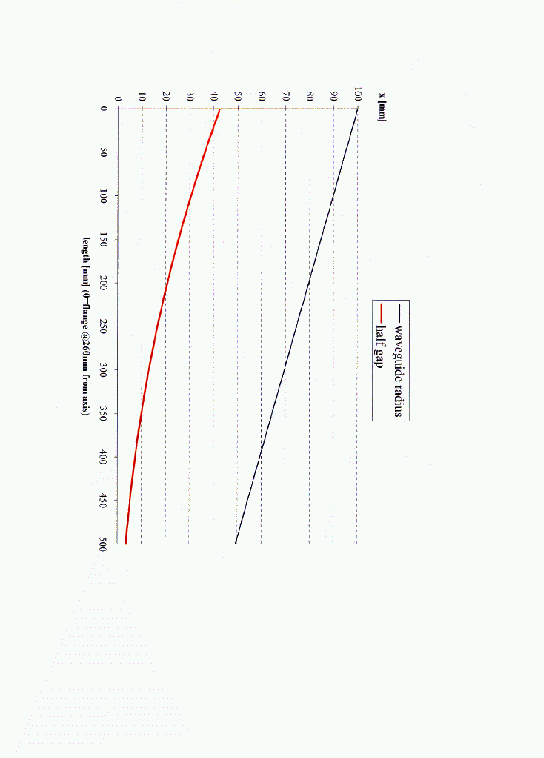## Macro 'Linear_Waveguide'

gd1 directly allows the modeling of cross-sections which vary as a linear function.
 macro Linear_Waveguide
define(zzPHI, @arg1 *@pi/180)     # First argument of the macro
define(ZPOSITION, @arg2)          # Second argument

define(GuideR0, @arg4)

-ggcylinder
material= 0
range= ( @arg3, @arg5 )

xprimedirection= ( cos(zzPHI+@pi/2), sin(zzPHI+@pi/2), 0 )
yprimedirection= ( 0, 0, 1 )
origin= ( 0, 0, ZPOSITION )

xslope= (@arg6/@arg4-1)/(@arg5-@arg3)
yslope= (@arg6/@arg4-1)/(@arg5-@arg3)

clear

point= ( GuideR0, 0 ) # x', y'
arc, radius= GuideR0, size= small, type= counterclockwise, deltaphi= 5
point= ( 0, GuideR0 )
arc,
point= ( -GuideR0, 0 )
arc,
point= ( 0, -GuideR0 )
arc,
point= ( GuideR0, 0 )

show= @arg7 # now|later|all
doit
endmacro

if (0) then
#
# for example, call the macro three times:
#
do Phi= 0, 240, 120
if (Phi == 240) then
sdefine(SHOW, all)
else
sdefine(SHOW, later)
endif
call Linear_Waveguide(Phi, \   # angle
10e-3, \ # z-position
260e-3, \           # starting position of the ggcylinder
100e-3, \           # start value outer radius
(260e-3+500e-3), \  # end position of the ggcylinder
50e-3, \            # endvalue of the outer radius
SHOW )
enddo
endif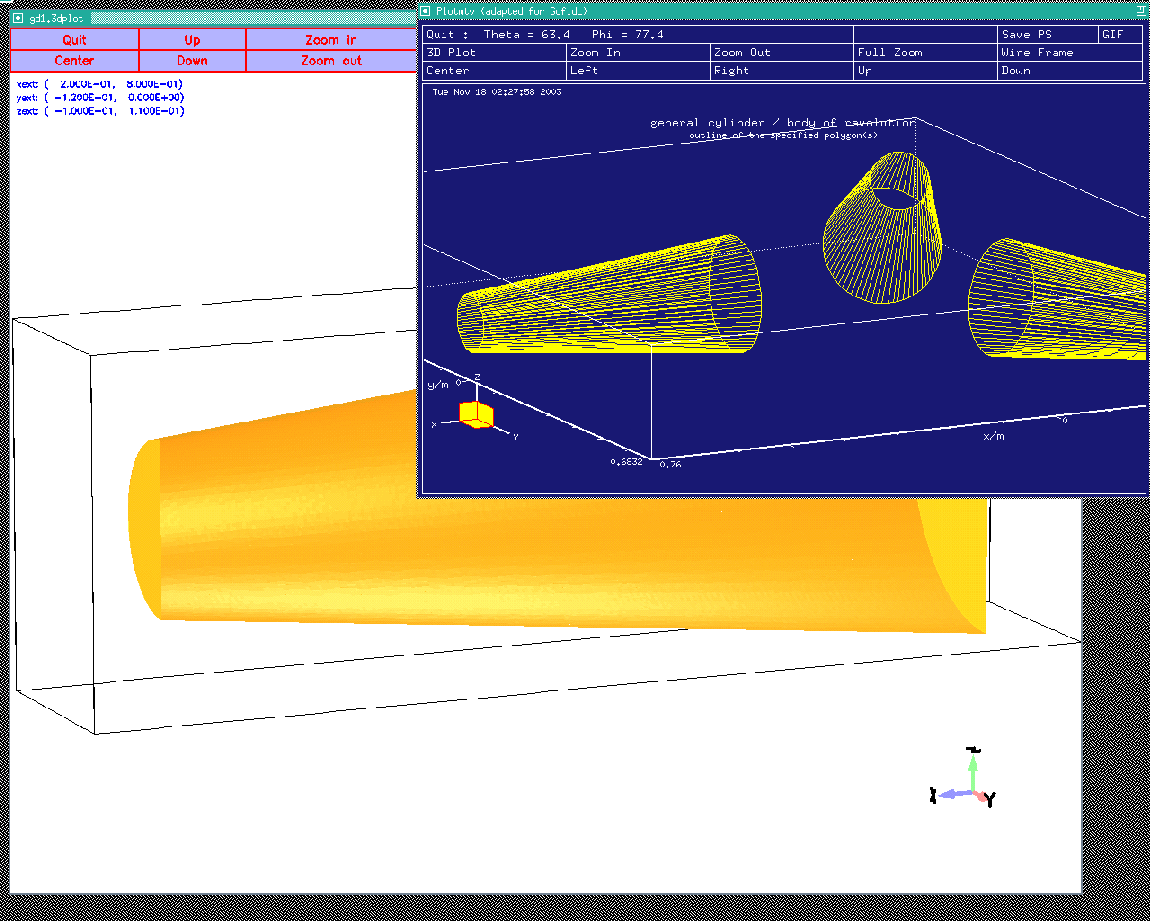## Macro 'Linear_Ridge'

The gap of the ridge varies nonlinear. We model the nonlinear behaviour by modeling the ridge as many short sections where the gap may be assumed varying linear.
 macro Linear_Ridge
define(zzPHI, @arg1 *@pi/180)     # First argument of the macro
define(ZPOSITION, @arg2)          # Second argument
define(GuideGap, 2*(@arg4) )      # @arg4 : Half starting gap

define(WW, 51e-3)
define(RR2, 2e-3)
define(RR5, 5e-3)

-ggcylinder
range= ( @arg3, @arg5 )

xprimedirection= ( cos(zzPHI+@pi/2), sin(zzPHI+@pi/2), 0 )
yprimedirection= ( 0, 0, 1 )
origin= ( 0, 0, ZPOSITION )

#
# We first refill a larger part with
# electric conducting material.
#
clear
point= ( -WW/2, -100e-3 ) # x', y'
point= (  WW/2, -100e-3 )
point= (  WW/2,  100e-3 )
point= ( -WW/2,  100e-3 )
material= 3
show= later
xslope= 0, yslope= 0
doit

#
# We now carve out the vacuum part
#
material= 0
xslope= 0
yslope= (@arg6/@arg4-1)/(@arg5-@arg3)

define(AA, WW/2+RR5)
define(BB, ((GuideR0-RR5)**2 - AA**2)**0.5)
define(THETA, atan2(BB, AA))

clear

# +x, +y
point= (  WW/2   , GuideGap/2+ RR2 )
arc, radius= RR2, size= small, type= clockwise
point= (  WW/2-RR2 , GuideGap/2 )

# -x, +y
point= ( -(WW/2-RR2), GuideGap/2 )
arc, radius= RR2, size= small, type= clockwise
point= ( -WW/2   , GuideGap/2+ RR2 )

# -x, -y
point= (  -WW/2   , -(GuideGap/2+ RR2) )
arc, radius= RR2, size= small, type= clockwise
point= (  -(WW/2-RR2), -GuideGap/2 )

# +x, -y
point= (  (WW/2-RR2), -GuideGap/2 )
arc, radius= RR2, size= small, type= clockwise
point= (  WW/2   , -(GuideGap/2+ RR2) )

show= @arg7 # now|later|all
doit
endmacro

#
# Call the macro for example like this:
#
if (0) then
call Linear_Ridge(0, \   # angle
10e-3, \ # z-position
260e-3, \           # starting position of the ggcylinder
43e-3, \           # start value half gap
(260e-3+500e-3), \  # end position of the ggcylinder
9e-3, \            # endvalue of half gap
all )
endif

When we call the macro after the linear varying waveguide has been modeled, we get a plot similiar to the one shown in figure 2.3. The used inputfile is:
 -brick
material= 3
volume= ( -INF,INF, -INF,INF, -INF,INF )
doit

include( macro-Linear_Waveguide.gdf )
include( macro-Linear_Ridge.gdf )

#
# Call the macros three times:
#
do Phi= 0, 240, 120
if (Phi == 240) then
sdefine(SHOW, all)
else
sdefine(SHOW, later)
endif
call Linear_Waveguide(Phi, \   # angle
10e-3, \ # z-position
260e-3, \           # starting position of the ggcylinder
100e-3, \           # start value outer radius
(260e-3+500e-3), \  # end position of the ggcylinder
50e-3, \            # endvalue of the outer radius
later )

call Linear_Ridge(Phi, \   # angle
10e-3, \ # z-position
260e-3, \           # starting position of the ggcylinder
43e-3, \           # start value half gap
(260e-3+500e-3), \  # end position of the ggcylinder
9e-3, \            # endvalue of half gap
SHOW )
enddo

-mesh
perfectmesh= yes
spacing= 2e-3
pxlow= 0.2, pxhigh= 0.8
pylow= -0.12, pyhigh= 0
pzlow= -0.1, pzhigh= 0.11

-volumeplot
doit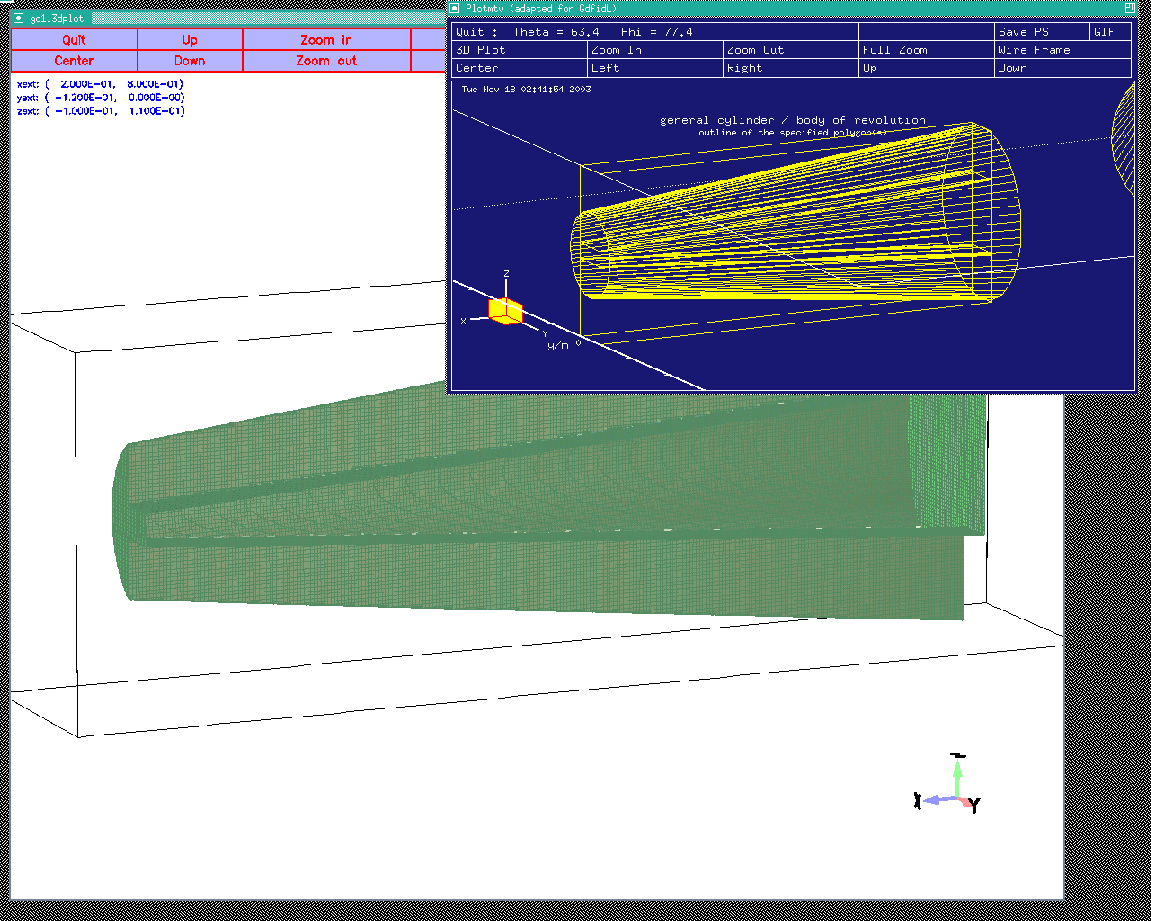Since the real ridgevaries nonlinearly, we now have to call the macro many times with the proper start and end values. The wanted gap-widths as a function position can be taken from the dataset provided by Dr Marhauser:

1. Waveguide Position
3. Half Gap-distance
5. Distance measured from Cavity-center
0       100             42.548  115     375
10      98.98448        41.2529 125     385
20      97.96896        39.9786 135     395
30      96.95344        38.7253 145     405
40      95.93792        37.4932 155     415
50      94.9224         36.2822 165     425
60      93.90688        35.0925 175     435
70      92.89136        33.9242 185     445
80      91.87584        32.7772 195     455
90      90.86032        31.6517 205     465
100     89.8448         30.5478 215     475
110     88.82928        29.4654 225     485
120     87.81376        28.4046 235     495
130     86.79824        27.3812 245     505
140     85.78272        26.3479 255     515
150     84.76720        25.3521 265     525
160     83.75168        24.378  275     535
170     82.73616        23.4255 285     545
180     81.72064        22.4947 295     555
190     80.70512        21.5856 305     565
200     79.68960        20.6981 315     575
210     78.67408        19.8322 325     585
220     77.65856        18.9879 335     595
230     76.64304        18.165  345     605
240     75.62752        17.3635 355     615
250     74.61200        16.5834 365     625
260     73.59648        15.8246 375     635
270     72.58096        15.0868 385     645
280     71.56544        14.3701 395     655
290     70.54992        13.6743 405     665
300     69.53440        12.9992 415     675
310     68.51888        12.3448 425     685
320     67.50336        11.7107 435     695
330     66.48784        11.0969 445     705
340     65.47232        10.5031 455     715
350     64.45680        9.92909 465     725
360     63.44128        9.3747  475     735
370     62.42576        8.83965 485     745
380     61.41024        8.32367 495     755
390     60.39472        7.82648 505     765
400     59.37920        7.3478  515     775
410     58.36368        6.88729 525     785
420     57.34816        6.44464 535     795
430     56.33264        6.01949 545     805
440     55.31712        5.61147 555     815
450     54.30160        5.22019 565     825
460     53.28608        4.84526 575     835
470     52.27056        4.48623 585     845
480     51.25504        4.14267 595     855
490     50.23952        3.8141  605     865
500     49.22400        3.5000  615     875

We can generate the needed calls to our function via a short program tha reads this dataset and then writes the appropriate file:
 ! This is a small Fortran program which generates
! the appropriate calls.

DO i= 1, 5
READ (*,*) ! Skip the first five lines
ENDDO

DO
WRITE (*,50) DistanceCenter*1e-3, HGap*1e-3
WRITE (*,60) DistanceCenter*1e-3
WRITE (*,70)                  HGap*1e-3
ENDDO

50 FORMAT(' call Linear_Ridge(Phi, ZPOS,', &
G17.7,', ', G17.7,', POS_OLD, G_OLD, later )')
60 FORMAT(' ')
70 FORMAT(' ')

END

The inputfile with the automaticalls generated calls:
 -brick
material= 3
volume= ( -INF,INF, -INF,INF, -INF,INF )
doit

include( macro-Linear_Waveguide.gdf )
include( macro-Linear_Ridge.gdf )

#
# Call the macros three times:
#
do Phi= 0, 240, 120
if (Phi == 240) then
sdefine(SHOW, all)
else
sdefine(SHOW, later)
endif
define(ZPOS, 10e-3)

#
#
call Linear_Waveguide(Phi, \   # angle
ZPOS, \ # z-position
200e-3, \           # starting position of the ggcylinder
100e-3, \           # start value outer radius
375e-3, \  # end position of the ggcylinder
100e-3, \            # endvalue of the outer radius
later )

#
# linear varying section:
#
call Linear_Waveguide(Phi, \   # angle
ZPOS, \ # z-position
375e-3, \           # starting position of the ggcylinder
100e-3, \           # start value outer radius
(375e-3+500e-3), \  # end position of the ggcylinder
50e-3, \            # endvalue of the outer radius
later )

define(POS_OLD,    0.200000     ) # inserted by hand
define(G_OLD,    0.4254800E-01 )  # inserted by hand
#
# The automatically generated part of the inputfile:
#
call Linear_Ridge(Phi, ZPOS,    0.2600000    ,     0.4254800E-01, POS_OLD, G_OLD, later )
define(POS_OLD,    0.2600000     )
define(G_OLD,    0.4254800E-01 )
call Linear_Ridge(Phi, ZPOS,    0.2700000    ,     0.4125290E-01, POS_OLD, G_OLD, later )
define(POS_OLD,    0.2700000     )
define(G_OLD,    0.4125290E-01 )
call Linear_Ridge(Phi, ZPOS,    0.2800000    ,     0.3997860E-01, POS_OLD, G_OLD, later )
define(POS_OLD,    0.2800000     )
define(G_OLD,    0.3997860E-01 )
call Linear_Ridge(Phi, ZPOS,    0.2900000    ,     0.3872530E-01, POS_OLD, G_OLD, later )
define(POS_OLD,    0.2900000     )
define(G_OLD,    0.3872530E-01 )
call Linear_Ridge(Phi, ZPOS,    0.3000000    ,     0.3749320E-01, POS_OLD, G_OLD, later )
define(POS_OLD,    0.3000000     )
define(G_OLD,    0.3749320E-01 )
call Linear_Ridge(Phi, ZPOS,    0.3100000    ,     0.3628220E-01, POS_OLD, G_OLD, later )
define(POS_OLD,    0.3100000     )
define(G_OLD,    0.3628220E-01 )
call Linear_Ridge(Phi, ZPOS,    0.3200000    ,     0.3509250E-01, POS_OLD, G_OLD, later )
define(POS_OLD,    0.3200000     )
define(G_OLD,    0.3509250E-01 )
call Linear_Ridge(Phi, ZPOS,    0.3300000    ,     0.3392420E-01, POS_OLD, G_OLD, later )
define(POS_OLD,    0.3300000     )
define(G_OLD,    0.3392420E-01 )
call Linear_Ridge(Phi, ZPOS,    0.3400000    ,     0.3277720E-01, POS_OLD, G_OLD, later )
define(POS_OLD,    0.3400000     )
define(G_OLD,    0.3277720E-01 )
call Linear_Ridge(Phi, ZPOS,    0.3500000    ,     0.3165170E-01, POS_OLD, G_OLD, later )
define(POS_OLD,    0.3500000     )
define(G_OLD,    0.3165170E-01 )
call Linear_Ridge(Phi, ZPOS,    0.3600000    ,     0.3054780E-01, POS_OLD, G_OLD, later )
define(POS_OLD,    0.3600000     )
define(G_OLD,    0.3054780E-01 )
call Linear_Ridge(Phi, ZPOS,    0.3700000    ,     0.2946540E-01, POS_OLD, G_OLD, later )
define(POS_OLD,    0.3700000     )
define(G_OLD,    0.2946540E-01 )
call Linear_Ridge(Phi, ZPOS,    0.3800000    ,     0.2840460E-01, POS_OLD, G_OLD, later )
define(POS_OLD,    0.3800000     )
define(G_OLD,    0.2840460E-01 )
call Linear_Ridge(Phi, ZPOS,    0.3900000    ,     0.2738120E-01, POS_OLD, G_OLD, later )
define(POS_OLD,    0.3900000     )
define(G_OLD,    0.2738120E-01 )
call Linear_Ridge(Phi, ZPOS,    0.4000000    ,     0.2634790E-01, POS_OLD, G_OLD, later )
define(POS_OLD,    0.4000000     )
define(G_OLD,    0.2634790E-01 )
call Linear_Ridge(Phi, ZPOS,    0.4100000    ,     0.2535210E-01, POS_OLD, G_OLD, later )
define(POS_OLD,    0.4100000     )
define(G_OLD,    0.2535210E-01 )
call Linear_Ridge(Phi, ZPOS,    0.4200000    ,     0.2437800E-01, POS_OLD, G_OLD, later )
define(POS_OLD,    0.4200000     )
define(G_OLD,    0.2437800E-01 )
call Linear_Ridge(Phi, ZPOS,    0.4300000    ,     0.2342550E-01, POS_OLD, G_OLD, later )
define(POS_OLD,    0.4300000     )
define(G_OLD,    0.2342550E-01 )
call Linear_Ridge(Phi, ZPOS,    0.4400000    ,     0.2249470E-01, POS_OLD, G_OLD, later )
define(POS_OLD,    0.4400000     )
define(G_OLD,    0.2249470E-01 )
call Linear_Ridge(Phi, ZPOS,    0.4500000    ,     0.2158560E-01, POS_OLD, G_OLD, later )
define(POS_OLD,    0.4500000     )
define(G_OLD,    0.2158560E-01 )
call Linear_Ridge(Phi, ZPOS,    0.4600000    ,     0.2069810E-01, POS_OLD, G_OLD, later )
define(POS_OLD,    0.4600000     )
define(G_OLD,    0.2069810E-01 )
call Linear_Ridge(Phi, ZPOS,    0.4700000    ,     0.1983220E-01, POS_OLD, G_OLD, later )
define(POS_OLD,    0.4700000     )
define(G_OLD,    0.1983220E-01 )
call Linear_Ridge(Phi, ZPOS,    0.4800000    ,     0.1898790E-01, POS_OLD, G_OLD, later )
define(POS_OLD,    0.4800000     )
define(G_OLD,    0.1898790E-01 )
call Linear_Ridge(Phi, ZPOS,    0.4900000    ,     0.1816500E-01, POS_OLD, G_OLD, later )
define(POS_OLD,    0.4900000     )
define(G_OLD,    0.1816500E-01 )
call Linear_Ridge(Phi, ZPOS,    0.5000000    ,     0.1736350E-01, POS_OLD, G_OLD, later )
define(POS_OLD,    0.5000000     )
define(G_OLD,    0.1736350E-01 )
call Linear_Ridge(Phi, ZPOS,    0.5100001    ,     0.1658340E-01, POS_OLD, G_OLD, later )
define(POS_OLD,    0.5100001     )
define(G_OLD,    0.1658340E-01 )
call Linear_Ridge(Phi, ZPOS,    0.5200000    ,     0.1582460E-01, POS_OLD, G_OLD, later )
define(POS_OLD,    0.5200000     )
define(G_OLD,    0.1582460E-01 )
call Linear_Ridge(Phi, ZPOS,    0.5300000    ,     0.1508680E-01, POS_OLD, G_OLD, later )
define(POS_OLD,    0.5300000     )
define(G_OLD,    0.1508680E-01 )
call Linear_Ridge(Phi, ZPOS,    0.5400000    ,     0.1437010E-01, POS_OLD, G_OLD, later )
define(POS_OLD,    0.5400000     )
define(G_OLD,    0.1437010E-01 )
call Linear_Ridge(Phi, ZPOS,    0.5500000    ,     0.1367430E-01, POS_OLD, G_OLD, later )
define(POS_OLD,    0.5500000     )
define(G_OLD,    0.1367430E-01 )
call Linear_Ridge(Phi, ZPOS,    0.5600000    ,     0.1299920E-01, POS_OLD, G_OLD, later )
define(POS_OLD,    0.5600000     )
define(G_OLD,    0.1299920E-01 )
call Linear_Ridge(Phi, ZPOS,    0.5700001    ,     0.1234480E-01, POS_OLD, G_OLD, later )
define(POS_OLD,    0.5700001     )
define(G_OLD,    0.1234480E-01 )
call Linear_Ridge(Phi, ZPOS,    0.5800000    ,     0.1171070E-01, POS_OLD, G_OLD, later )
define(POS_OLD,    0.5800000     )
define(G_OLD,    0.1171070E-01 )
call Linear_Ridge(Phi, ZPOS,    0.5900000    ,     0.1109690E-01, POS_OLD, G_OLD, later )
define(POS_OLD,    0.5900000     )
define(G_OLD,    0.1109690E-01 )
call Linear_Ridge(Phi, ZPOS,    0.6000000    ,     0.1050310E-01, POS_OLD, G_OLD, later )
define(POS_OLD,    0.6000000     )
define(G_OLD,    0.1050310E-01 )
call Linear_Ridge(Phi, ZPOS,    0.6100000    ,     0.9929090E-02, POS_OLD, G_OLD, later )
define(POS_OLD,    0.6100000     )
define(G_OLD,    0.9929090E-02 )
call Linear_Ridge(Phi, ZPOS,    0.6200000    ,     0.9374700E-02, POS_OLD, G_OLD, later )
define(POS_OLD,    0.6200000     )
define(G_OLD,    0.9374700E-02 )
call Linear_Ridge(Phi, ZPOS,    0.6300001    ,     0.8839651E-02, POS_OLD, G_OLD, later )
define(POS_OLD,    0.6300001     )
define(G_OLD,    0.8839651E-02 )
call Linear_Ridge(Phi, ZPOS,    0.6400000    ,     0.8323670E-02, POS_OLD, G_OLD, later )
define(POS_OLD,    0.6400000     )
define(G_OLD,    0.8323670E-02 )
call Linear_Ridge(Phi, ZPOS,    0.6500000    ,     0.7826480E-02, POS_OLD, G_OLD, later )
define(POS_OLD,    0.6500000     )
define(G_OLD,    0.7826480E-02 )
call Linear_Ridge(Phi, ZPOS,    0.6600000    ,     0.7347800E-02, POS_OLD, G_OLD, later )
define(POS_OLD,    0.6600000     )
define(G_OLD,    0.7347800E-02 )
call Linear_Ridge(Phi, ZPOS,    0.6700000    ,     0.6887290E-02, POS_OLD, G_OLD, later )
define(POS_OLD,    0.6700000     )
define(G_OLD,    0.6887290E-02 )
call Linear_Ridge(Phi, ZPOS,    0.6800000    ,     0.6444640E-02, POS_OLD, G_OLD, later )
define(POS_OLD,    0.6800000     )
define(G_OLD,    0.6444640E-02 )
call Linear_Ridge(Phi, ZPOS,    0.6900001    ,     0.6019490E-02, POS_OLD, G_OLD, later )
define(POS_OLD,    0.6900001     )
define(G_OLD,    0.6019490E-02 )
call Linear_Ridge(Phi, ZPOS,    0.7000000    ,     0.5611470E-02, POS_OLD, G_OLD, later )
define(POS_OLD,    0.7000000     )
define(G_OLD,    0.5611470E-02 )
call Linear_Ridge(Phi, ZPOS,    0.7100000    ,     0.5220190E-02, POS_OLD, G_OLD, later )
define(POS_OLD,    0.7100000     )
define(G_OLD,    0.5220190E-02 )
call Linear_Ridge(Phi, ZPOS,    0.7200000    ,     0.4845260E-02, POS_OLD, G_OLD, later )
define(POS_OLD,    0.7200000     )
define(G_OLD,    0.4845260E-02 )
call Linear_Ridge(Phi, ZPOS,    0.7300000    ,     0.4486230E-02, POS_OLD, G_OLD, later )
define(POS_OLD,    0.7300000     )
define(G_OLD,    0.4486230E-02 )
call Linear_Ridge(Phi, ZPOS,    0.7400000    ,     0.4142670E-02, POS_OLD, G_OLD, later )
define(POS_OLD,    0.7400000     )
define(G_OLD,    0.4142670E-02 )
call Linear_Ridge(Phi, ZPOS,    0.7500001    ,     0.3814100E-02, POS_OLD, G_OLD, later )
define(POS_OLD,    0.7500001     )
define(G_OLD,    0.3814100E-02 )
call Linear_Ridge(Phi, ZPOS,    0.7600001    ,     0.3500000E-02, POS_OLD, G_OLD, SHOW )
define(POS_OLD,    0.7600001     )
define(G_OLD,    0.3500000E-02 )

enddo

-mesh
perfectmesh= yes
spacing= 2e-3
pxlow= 0.19, pxhigh= 0.8
pylow= -0.12, pyhigh= 0
pzlow= -0.1, pzhigh= 0.11

-volumeplot
doit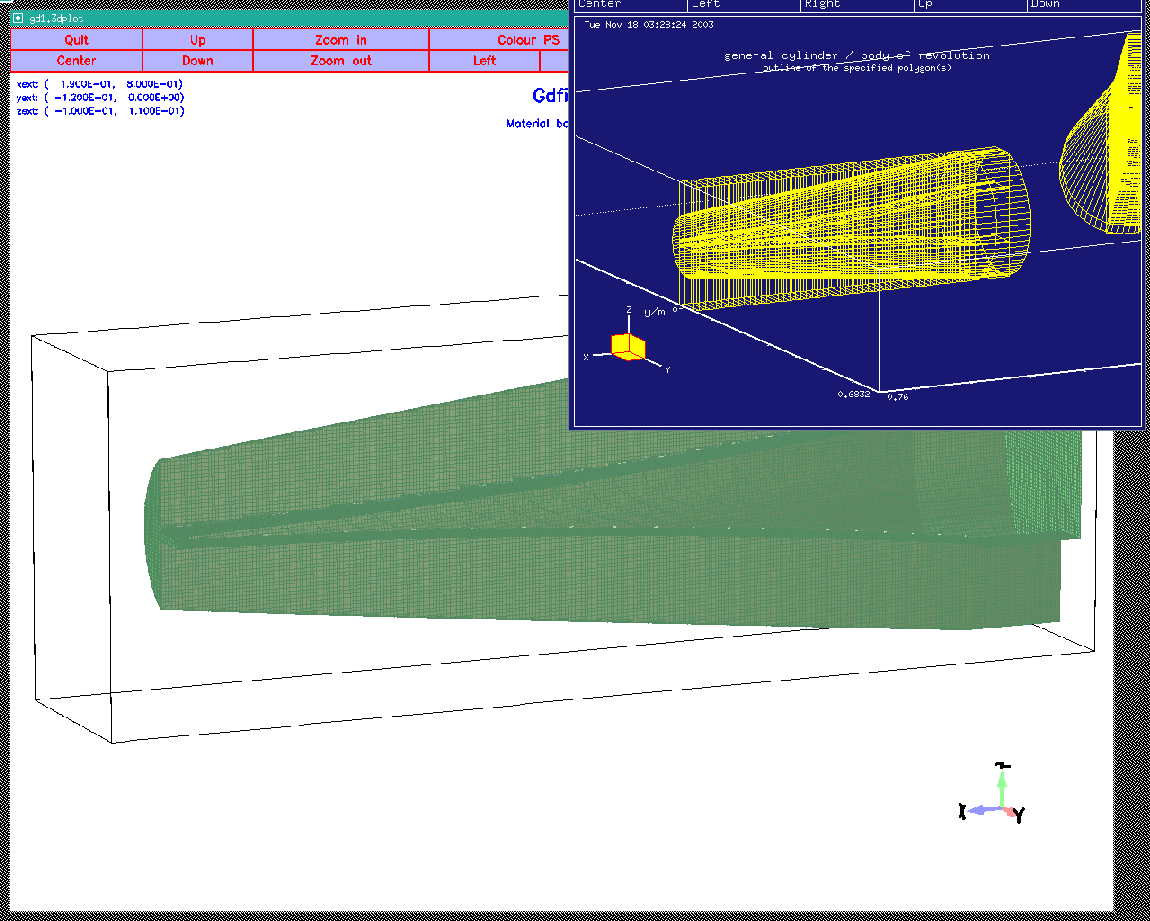# Modeling the transformer and the coaxial waveguides

The transformer can be modeled by filling cylinders with a general footprint near the end of the varying waveguide.
 macro transformer
define(zzPHI, (@arg1)*@pi/180 )
define(ZOFF, @arg2)

define(RALT, 50e-3) # The last radius
-ggcyl
material= 1
xprime= ( cos(zzPHI      ), sin(zzPHI      ), 0 )
yprime= ( cos(zzPHI+@pi/2), sin(zzPHI+@pi/2), 0 )
xslope= 0, yslope= 0
define(RR, 906.0e-3 - 31e-3)
echo *** r-Position of tip of ellipse: eval(RR+31e-3) ( should be 906.0e-3 )
origin= ( RR*cos(zzPHI), RR*sin(zzPHI), ZOFF )
clear
point= ( 0, -51e-3/2 )
ellipse, center= ( 0, 0 ), size= small
point= ( 31e-3, 0 )
ellipse
point= ( 0,  51e-3/2 )
range= ( 3.5e-3, RALT )
show= later
doit
range= ( -RALT, -3.5e-3 )
show= @arg3
doit

endmacro

if (0) then
#
# Call this macro eg like this:
#
call transformer(0, 0, later )
endif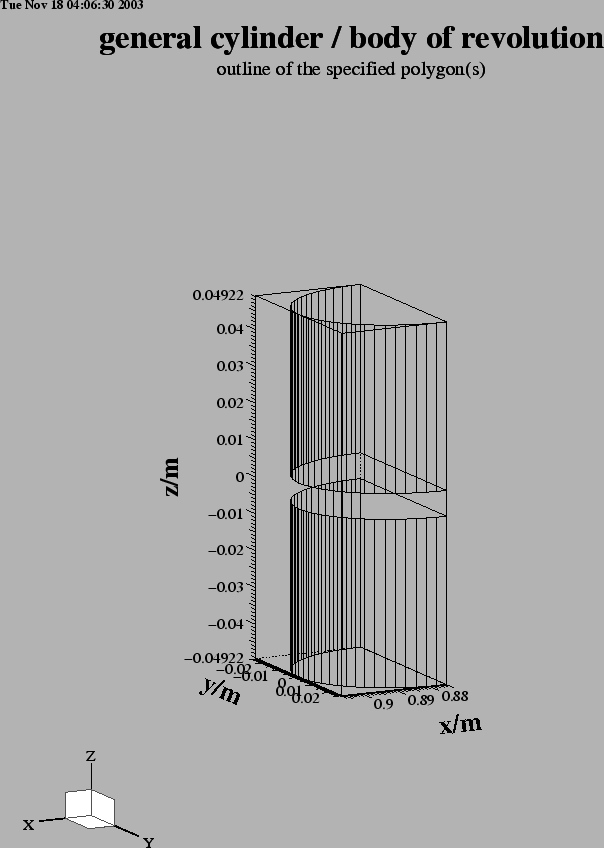The coaxial waveguides are easily described as circular cylinders. In the first step, a circular cylinder is carved out with a radius of the outer conductor. In the second step, the volume of the inner conductor is refilled. We add these cylinders to the previous macro and get:

 macro transformer_and_coaxial
define(zzPHI, (@arg1)*@pi/180 )
define(ZOFF, @arg2)

define(RALT, 50e-3) # The last radius
-ggcyl
material= 3
xprime= ( cos(zzPHI      ), sin(zzPHI      ), 0 )
yprime= ( cos(zzPHI+@pi/2), sin(zzPHI+@pi/2), 0 )
xslope= 0, yslope= 0
define(RR, 906.0e-3 - 31e-3)
echo *** r-Position of tip of ellipse: eval(RR+31e-3) ( should be 906.0e-3 )
origin= ( RR*cos(zzPHI), RR*sin(zzPHI), ZOFF )
clear
point= ( 0, -51e-3/2 )
ellipse, center= ( 0, 0 ), size= small
point= ( 31e-3, 0 )
ellipse
point= ( 0,  51e-3/2 )
range= ( 3.5e-3, RALT )
show= later
doit
range= ( -RALT, -3.5e-3 )
show= @arg3
doit

#
# The coaxial line:
#
### r-Position of coaxial lines:
define(RR, 894.2e-3-19.2e-3)

define(XOFF, (RR+19.2e-3)*cos(zzPHI) )
define(YOFF, (RR+19.2e-3)*sin(zzPHI) )

-mesh
zfixed( 2, -3.5e-3+ZOFF, 3.5+ZOFF )
xfixed( 3, XOFF-19.4e-3, XOFF- 8.4e-3 )
yfixed( 3, YOFF-19.4e-3, YOFF- 8.4e-3 )
xfixed( 3, XOFF+ 8.4e-3, XOFF+19.4e-3 )
yfixed( 3, YOFF+ 8.4e-3, YOFF+19.4e-3 )

-gccylinder
length= INF
origin= ( XOFF, YOFF, ZOFF-3.5e-3 )
direction= ( 0, 0, 1 )
material= 0, radius= 19.4e-3/2, doit   # Carve out radius of outer conductor

origin= ( XOFF, YOFF, ZOFF-4.5e-3 )
material= 4, radius=  8.4e-3/2, doit   # Refill inner conductor

endmacro

# call transformer_and_coaxial(Phi, Zpos, later)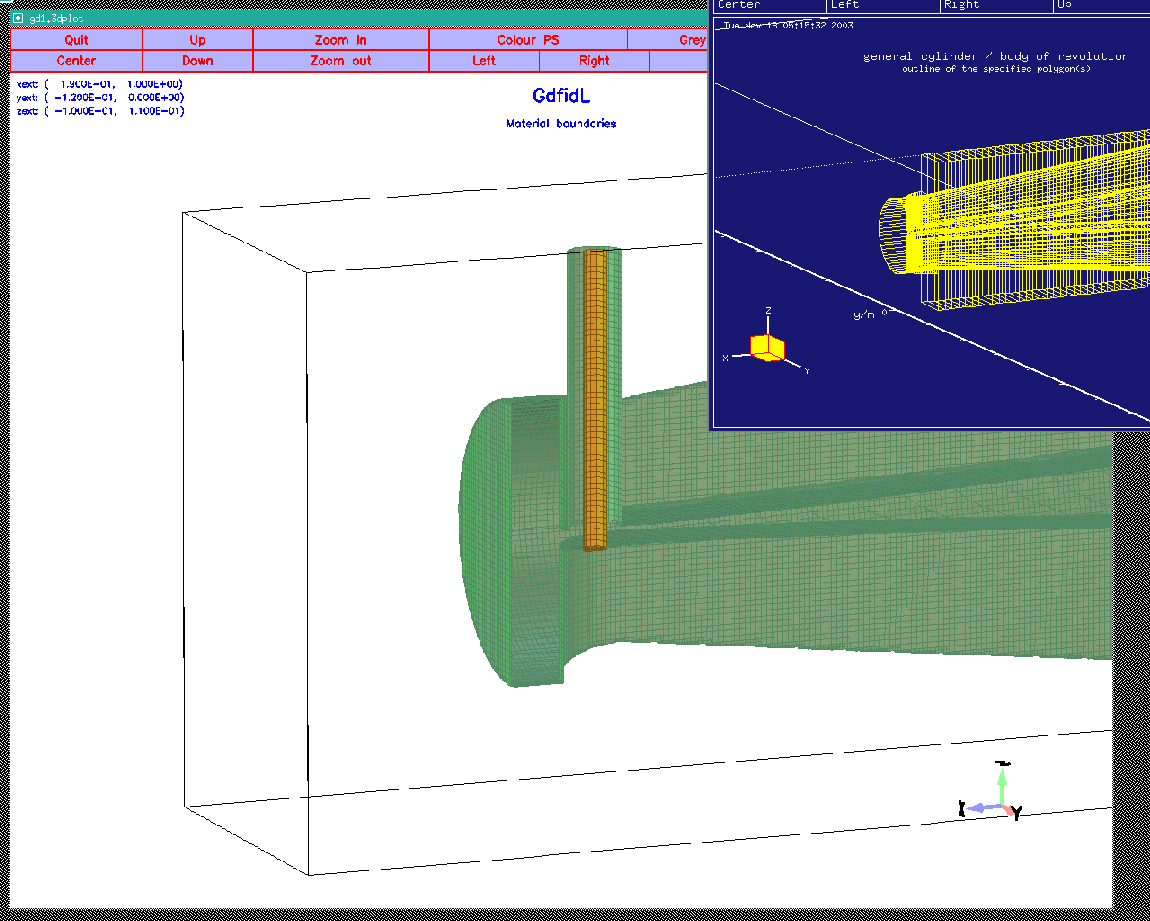# Putting it all together

We are now in the position to but it all together. The steps to model the geometry are: Fill the whole computational volume with vacuum, then carve out the waveguides, then carve out the cavity itself. As a last step, the ports need to be defined. If we do not use the symmetry plane at y=0 (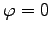), we have four ports ,the beam pipe and the three coaxial lines, at the upper z-boundary of the computational volume, and one port, the lower beam pipe, at the lower z-boundary.
 -general

dice= yes   # If computing in parallel, use the subvolume algorithm
##    ndpw= 24    # use 24 subvolumes per CPU
iodice= yes # store the results on the local discs of the nodes

-material
material= 3, type= electric
material= 4, type= electric

define(STPSZE, 2e-3)
-mesh
spacing= STPSZE

#
# The borders of the computational volume:
#
define(YLOW, -0.94)
define(YHIGH, 0)
pxlow= -0.6, pxhigh= 1.0
pylow= YLOW, pyhigh= YHIGH
pzlow= -0.2, pzhigh= 0.2

#
# The conditions at the borders:
#
cxlow= electric, cxhigh= electric
cylow= electric, cyhigh= magnetic
czlow= electric, czhigh= electric

-brick
material= 3
volume= ( -INF,INF, -INF,INF, -INF,INF )
doit

include( macro-Linear_Waveguide.gdf )
include( macro-Linear_Ridge.gdf )
include( macro-transformer-and-coaxial.gdf )

#
# Call the macros three times:
#
do iPhi= 1, 3
if (iPhi == 3) then
sdefine(SHOW, all)
else
sdefine(SHOW, later)
endif
if (iPhi == 1) then
define(ZPOS, 0.0495)
else
define(ZPOS, -0.0495)
endif

define(Phi, 0+(iPhi-1)*120)

#
# constant radius section of the circular part:
#
call Linear_Waveguide(Phi, \   # angle
ZPOS, \  # z-position
170e-3, \   # starting position of the ggcylinder
100e-3, \   # start value outer radius
375e-3, \   # end position of the ggcylinder
100e-3, \   # endvalue of the outer radius
later )

#
# constant section of the ridge:
#
call Linear_Ridge(Phi, \   # angle
ZPOS, \  # z-position
200e-3, \    # starting position of the ggcylinder
43e-3, \     # start value half gap
375e-3, \    # end position of the ggcylinder
9e-3, \      # endvalue of half gap
later )

#
# linear varying section:
#
call Linear_Waveguide(Phi, \   # angle
ZPOS, \ # z-position
375e-3, \  # starting position of the ggcylinder
100e-3, \  # start value outer radius
875e-3, \  # end position of the ggcylinder
50e-3, \   # endvalue of the outer radius
later )

#
# constant radius section, the part where the transformer is in
#
call Linear_Waveguide(Phi, \   # angle
ZPOS, \ # z-position
875e-3, \        # starting position of the ggcylinder
50e-3, \         # start value outer radius
(875e-3+65e-3), \  # end position of the ggcylinder
50e-3, \            # endvalue of the outer radius
later )

#
# Model the non-linear ridge via the many calls:
#
include( non-linear-ridge.gdf )

#
# Model the transformer and the coaxial line:
#
call transformer_and_coaxial(Phi, ZPOS, SHOW )

#
# tell GdfidL that the coaxial lines
# shall be treated as ports.
#
define(XX, 900e-3*cos(Phi*@pi/180) )
define(YY, 900e-3*sin(Phi*@pi/180) )
if (YY+50e-3 >= YLOW) then
if (YY-50e-3 <= YHIGH) then
#
# Only specify the port
# if at least a part of it
# is really within the computational volume
#
-fdtd
-ports
name= coax-phi=Phi, plane= zhigh, npml= 20, modes= 1
pxlow= XX-50e-3, pxhigh= XX+50e-3
pylow= YY-50e-3, pyhigh= YY+50e-3
doit
endif
endif

enddo # iPhi-loop

#
# tell GdfidL that the beam-pipes
# shall be treated as ports.
#
-fdtd
-ports
name= beamlow, plane= zlow, npml= 40, modes= 0
pxlow= -100e-3, pxhigh= 100e-3
pylow= -100e-3, pyhigh= 100e-3
doit

name= beamhigh, plane= zhigh, npml= 40, modes= 0
pxlow= -100e-3, pxhigh= 100e-3
pylow= -100e-3, pyhigh= 100e-3
doit

#
# Model the cavity itself:
#
include(Cavity.gdf)

-volumeplot
doit

#
# Specify that we want to perform a wakepotential-computation:
#
-fdtd
-lcharge
charge= 1e-12
xposition= 0
yposition= 0
sigma= 5*STPSZE
shigh= 400

#
# start the computation:
#
-fdtd
doit

This is the end of this tutorial.

#### Footnotes

... data1
from Dr Marhauser from Berliner Elektronen Synchrotron, BESSY